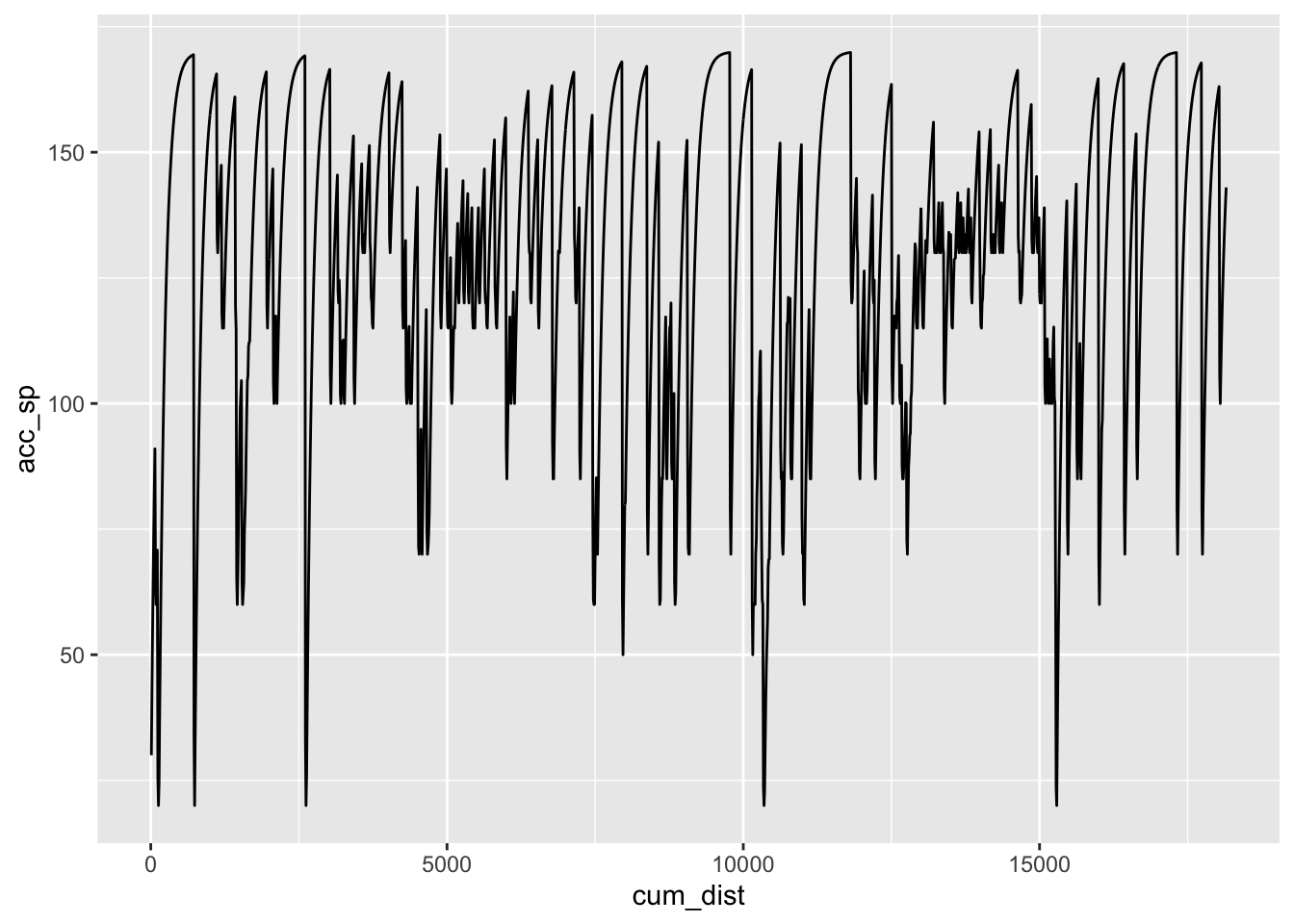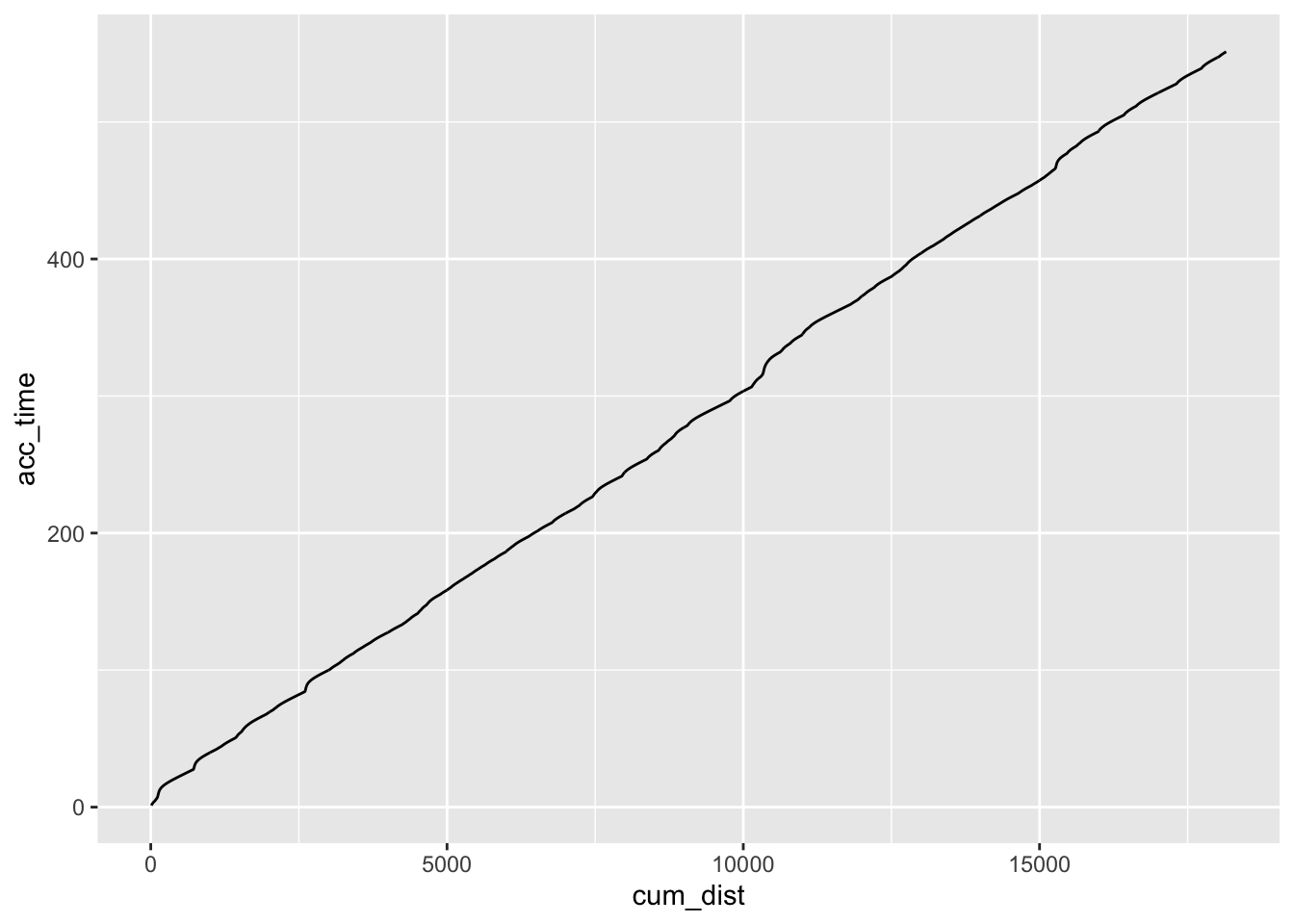# 10 Interpolation Using A Route Speed Model

Based on the curvature of the route, the speed model gives us a crude estimate of how long it takes to get between two points. If the route is a flat straight between the points, we’ll travel 1km in much less time than if the route it tight and twisty.

The simple `approxfun()` interpolator uses a linear model to interpolate times between actual time points, but we might be able to improve interpolated estimates using a non-linear model based on a speed model generated over the route based on the route curvature.

• identify distance into stage required (`desired_d`)
• find distance into stage for each
• get consecutive telemetry points between which desired distance lays (`in_d`, `out_d`, `in_t`, `out_t`)
``````> l = c(1.1,2.1,3.1,4.1,5.1)
> c(l[findInterval(3, l)], l[findInterval(3, l)+1])
 2.1 3.1
> c(l[findInterval(1, l)], l[findInterval(1, l)+1])
 1.1
> c(l[findInterval(11, l)], l[findInterval(11, l)+1])
 5.1  NA``````
• get speed model
• get model time at points (in_mt=model(in_d), out_mt=model(out_d), desired_mt=model(desired_d))
• get interpolated normalised model time between points, desired_nt = (desired_mt - in_mt)/(out_mt - in_mt)
• get predicted time desired_t = desired_nt*(out_t - in_t) + in_t

## 10.1 Creating a Route Speed Model

In the To See The Invisible rally pacenotes tutorial series by David Nafría, corners are given a particular severity based on curvature. Simple models of expected speeds for corners of a particular severity are also described for different classes of rally car.

We can use this approach to create a simple speed model based on curvature of the rally route. Using the expected cornering speed as a target speed and a simple acceleration model, as well as theoretical maximum speed, we can accelerate the car into and out of each corner based and generate a speed model as a result.

For more on analysing and visualising rally stage routes, see Visualising WRC Rally Stages With rayshader and R.

The `rLFT` processing linear features R package provides a handy tool for modeling curvature along each point of a route in the form of a boundary convexity tool (`bct()`). The curvature of a moving segment along the route is determined, along with the center of curvature and a convexity measure.

``````library(rLFT)

# The step dist is how far we move the window at each step
stepdist = 10
# The window is the length of the route for which we find the curvature
window = 20
get_route_convexity = function(route_basis_utm, stepdist=10, window=20){
bct(route_basis_utm,
# distance between measurements
step = stepdist,
window = window, ridName = "name") %>%
cum_dist = cumsum(dist))
}

route_convexity = get_route_convexity(stage_route_utm)``````
``````##    user  system elapsed
##   0.180   0.033   0.214
##  "Features skipped due to size: "
## logical(0)``````
``head(route_convexity, 3)``
``````##   FID                   RID MidMeas WindowSize RawConvexity ConvexityIndex
## 1   1 SS7/11 KakaristoHassi      10         20        0.000          0.000
## 2   1 SS7/11 KakaristoHassi      20         20        0.000          0.000
## 3   1 SS7/11 KakaristoHassi      30         20        0.788          0.079
##   Sinuosity Midpoint_X Midpoint_Y dist cum_dist
## 1     0.500   400689.0    6857086   10       10
## 2     0.500   400698.6    6857084   10       20
## 3     0.503   400708.3    6857081   10       30``````

Although the boundary convexity tool gives us a convexity measure, we can also create our own curvature metric that corresponds more closely to Nafría’s model. I don’t know how to write vectorised functions properly, so I’ll create a simple function that generates the curvature at a particular point on a route, and then use the `Vectorize()` helper function to as-if vectorise it for me.

``````library(devtools)
# The curvature function takes an arc defined over
# x and y coordinate lists

#circlefit, from pracma::
circlefit = function (xp, yp, fast = TRUE)
{
if (!is.vector(xp, mode = "numeric") || !is.vector(yp, mode = "numeric"))
stop("Arguments 'xp' and 'yp' must be numeric vectors.")
if (length(xp) != length(yp))
stop("Vectors 'xp' and 'yp' must be of the same length.")
if (!fast)
warning("Option 'fast' is deprecated and will not be used!",
call. = FALSE, immediate. = TRUE)
n <- length(xp)
p <- qr.solve(cbind(xp, yp, 1), matrix(xp^2 + yp^2, ncol = 1))
v <- c(p/2, p/2, sqrt((p^2 + p^2)/4 + p))
rms <- sqrt(sum((sqrt((xp - v)^2 + (yp - v)^2) - v)^2)/n)
#cat("RMS error:", rms, "\n")
return(v)
}

curvature = function(x,y){
#729181.8, 729186.1, 729190.4
#4957667 , 4957676, 4957685
tryCatch({
# circlefit gives an error if we pass a straight line
# Also hide the print statement in circlefit
# circlefit() returns the x and y coords of the circle center
# as well as the radius of curvature
# We could then also calculate the angle and arc length
circlefit(x,y)
},
error = function(err) {
# For a straight, return the first co-ord and Inf diameter
# Alternatively, pass zero diameter?
c(x, y, Inf)})
}

curvature2 = function(x1, x2, x3, y1, y2, y3){
curvature(c(x1, x2, x3), c(y1, y2, y3))
}

# The base::Vectorize function provides a lazy way of
# vectorising a non-vectorised function
curvatures_ = Vectorize(curvature2)

curvatures = function(route_convexity){
curvatures_(lag(route_convexity\$Midpoint_X),
route_convexity\$Midpoint_X,
lag(route_convexity\$Midpoint_Y),
route_convexity\$Midpoint_Y,
}``````

This model uses the corner centre measures calculated by the boundary convexity tool to return a radius for the curvature of each segment:

``route_convexity\$radius = curvatures(route_convexity)``

We can now generate the cornering speed model. The corner speed model generates several things:

• a corner index, `invisble_ci`, which is an integer representing the corner radius;
• a notional segment/corner target speed, `invisible_sp`.
``````corner_speed_model = function(route_convexity,
invisible_speeds = c(20, 40, 50, 60, 70, 85,
100, 115, 120, 130, 170),
speed_modifier = 0){

# Provide a simple means of increasing the cornering speeds
invisible_speeds = invisible_speeds + speed_modifier

invisible_bins = c(0, 10, 15, 20, 27.5, 35,
45, 60, 77.5, 100, 175, Inf)

breaks = invisible_bins,
labels = 1:(length(invisible_bins)-1),
ordered_result=TRUE)

# Speeds in km/h
#invisible_speeds = c(10, 40, 50, 60, 70, 80,
#                     95, 110, 120, 130, 180)

breaks = invisible_bins,
labels = invisible_speeds,
ordered_result=TRUE)

# Cast speed as factor, via character, to integer
route_convexity\$invisible_sp = as.integer(as.character(route_convexity\$invisible_sp))

route_convexity
}``````

Applying the speed model to our route gives us a corner index and notional target speed for each segment:

``````route_convexity = route_convexity %>% corner_speed_model()

``````##   FID                   RID MidMeas WindowSize RawConvexity ConvexityIndex
## 1   1 SS7/11 KakaristoHassi      10         20        0.000          0.000
## 2   1 SS7/11 KakaristoHassi      20         20        0.000          0.000
## 3   1 SS7/11 KakaristoHassi      30         20        0.788          0.079
##   Sinuosity Midpoint_X Midpoint_Y dist cum_dist radius invisible_ci
## 1     0.500   400689.0    6857086   10       10    Inf           11
## 2     0.500   400698.6    6857084   10       20    Inf           11
## 3     0.503   400708.3    6857081   10       30    Inf           11
##   invisible_sp
## 1          170
## 2          170
## 3          170``````

We can build up the speed model for the route. At each step we accelerate towards the nominal sector target speed (the `invisible_sp` value). We can’t accelerate infinitely fast, so our actual target accumulated speed for the segment, `acc_sp`, is a simple function of the current speed and the notional target speed. We can then calculate the notional time to complete that segment, `invisible_time`.

``````acceleration_model = function(route_convexity, stepdist=10,
acc = 0.1, dec = 0.1) {
# Acceleration model
sp = route_convexity\$invisible_sp
# Nominal starting target speed
# In we don't set this, we don't get started moving
sp = 30

# Use crude acceleration / brake weights
for (i in 2:(length(sp)-1)) {
# Simple linear model - accumulated speed is based on
# the current speed and the notional segment speed
# Accelerate up
if (sp[i-1]<=sp[i]) sp[i] = (sp[i-1] + acc * sp[i]) / (1+acc)

# Decelerate down
if (sp[i]>sp[i+1]) sp[i] = (dec * sp[i] + sp[i+1]) / (1+dec)
}

route_convexity\$acc_sp = sp
route_convexity\$acc_sp[length(sp)] = route_convexity\$invisible_sp[length(sp)]

# New time model
# Also get speed in m/s for time calculation
meters = 1000
seconds_per_hour = 3600 # 60 * 60
kph_unit = meters / seconds_per_hour
route_convexity = route_convexity %>%
mutate(segment_sp = route_convexity\$acc_sp * kph_unit,
invisible_time = dist/segment_sp,
acc_time = cumsum(invisible_time))

# So now we need to generate kilometer marks
route_convexity\$kmsection = 1 + trunc(route_convexity\$MidMeas/1000)
# We can use this to help find the time over each km

route_convexity
}``````

If we now apply the acceleration model to the route, we can calculate the speed over each segment, and the time taken to complete the segment:

``````route_convexity = acceleration_model(route_convexity)

``````##   FID                   RID MidMeas WindowSize RawConvexity ConvexityIndex
## 1   1 SS7/11 KakaristoHassi      10         20        0.000          0.000
## 2   1 SS7/11 KakaristoHassi      20         20        0.000          0.000
## 3   1 SS7/11 KakaristoHassi      30         20        0.788          0.079
##   Sinuosity Midpoint_X Midpoint_Y dist cum_dist radius invisible_ci
## 1     0.500   400689.0    6857086   10       10    Inf           11
## 2     0.500   400698.6    6857084   10       20    Inf           11
## 3     0.503   400708.3    6857081   10       30    Inf           11
##   invisible_sp   acc_sp segment_sp invisible_time acc_time kmsection
## 1          170 30.00000   8.333333      1.2000000 1.200000         1
## 2          170 42.72727  11.868687      0.8425532 2.042553         1
## 3          170 54.29752  15.082645      0.6630137 2.705567         1``````

Summing over the segment times (omitting the flying finish which has no specified ongoing segment length) gives us an estimated stage time

``````anticipated_time = function(route_convexity) {
anticipated_time = sum(route_convexity\$invisible_time[1:nrow(route_convexity)-1])
cat(paste0("Anticipated stage time: ", anticipated_time %/% 60,
'm ', round(anticipated_time %% 60, 1), 's' ))
}

anticipated_time(route_convexity)``````
``## Anticipated stage time: 9m 11.2s``

We can also view the speed model over distance into stage:

``ggplot(route_convexity) + geom_line(aes(x=cum_dist, y=acc_sp))``We can also plot the time model into the stage as the accumulated time:

``ggplot(route_convexity) + geom_line(aes(x=cum_dist, y=acc_time))``